EngliSea > P > physics

Classical Physics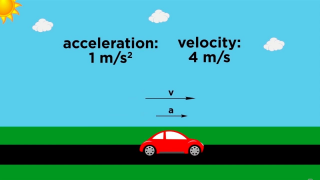03 Position/Velocity/Acceleration
Position/Velocity/Acceleration Part 1: Definitions
4789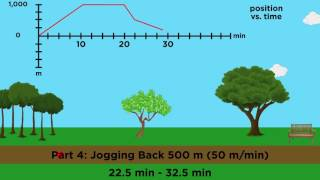04 Position/Velocity/Acceleration
Position/Velocity/Acceleration Part 2: Graphical Analysis
4790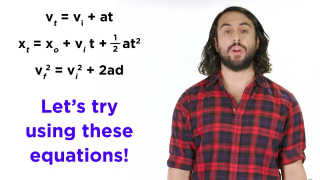05 Horizontal Motion
Kinematics Part 1: Horizontal Motion
480306 Vertical Motion
Kinematics Part 2: Vertical Motion
4804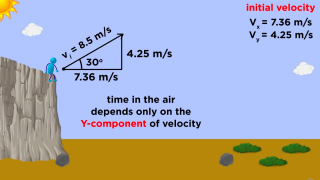07 Projectile Motion
Kinematics Part 3: Projectile Motion
4805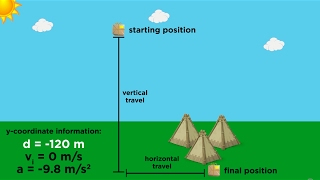08 Practice Problems and Strategy
Kinematics Part 4: Practice Problems and Strategy
4806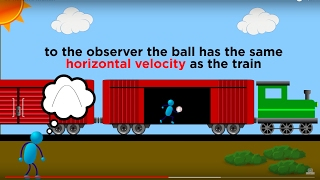09 Relative Motion and Inertial Reference Frames
Relative Motion and Inertial Reference Frames
4807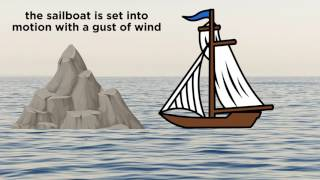11 Newton's First Law of Motion: Mass and Inertia
Newton's First Law of Motion: Mass and Inertia
4809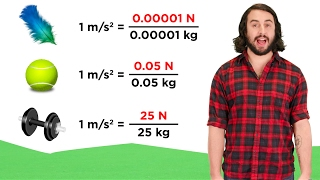12 Newton's Second Law of Motion
Newton's Second Law of Motion: F = ma
4810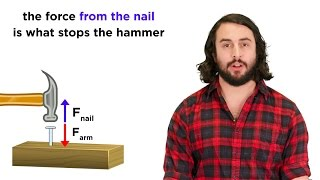13 Newton's Third Law of Motion: Action and Reaction
Newton's Third Law of Motion: Action and Reaction
4811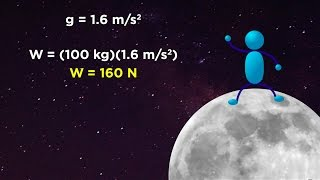14 Mass vs. Weight and the Normal Force
￫ inertia, parlance, appreciable, exert
4812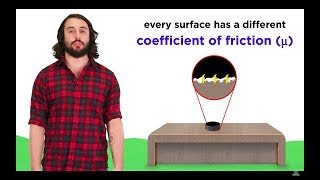15 Frictional Forces: Static and Kinetic
Frictional Forces: Static and Kinetic
4813Proverbs and Quotes in English
Short sentences and easy words.17 Kinetic Energy and Potential Energy
Kinetic Energy and Potential Energy
4815-